# Greater than Sign

Greater than Sign

## Table of Contents

 1 Introduction to the Greater than Sign 2 What is Greater than Sign? 3 Greater than Sign: Examples 4 Tips and Tricks 5 How to use Greater than Sign in Math? 6 Thinking out of the Box! 7 Practice Questions on Greater than Sign 9 Maths Olympiad Sample Papers 8 Frequently Asked Questions (FAQs)

We at Cuemath believe that Math is a life skill. Our Math Experts focus on the “Why” behind the “What.” Students can explore from a huge range of interactive worksheets, visuals, simulations, practice tests, and more to understand a concept in depth.

Book a FREE trial class today! and experience Cuemath’s LIVE Online Class with your child.

## Introduction to the Greater than Sign

In Mathematics, we use numbers for comparisons.

To compare means examining the difference between quantities or values to decide which is greater than, or equal to, or smaller than the other quantity.

We can do comparisons in terms of distances.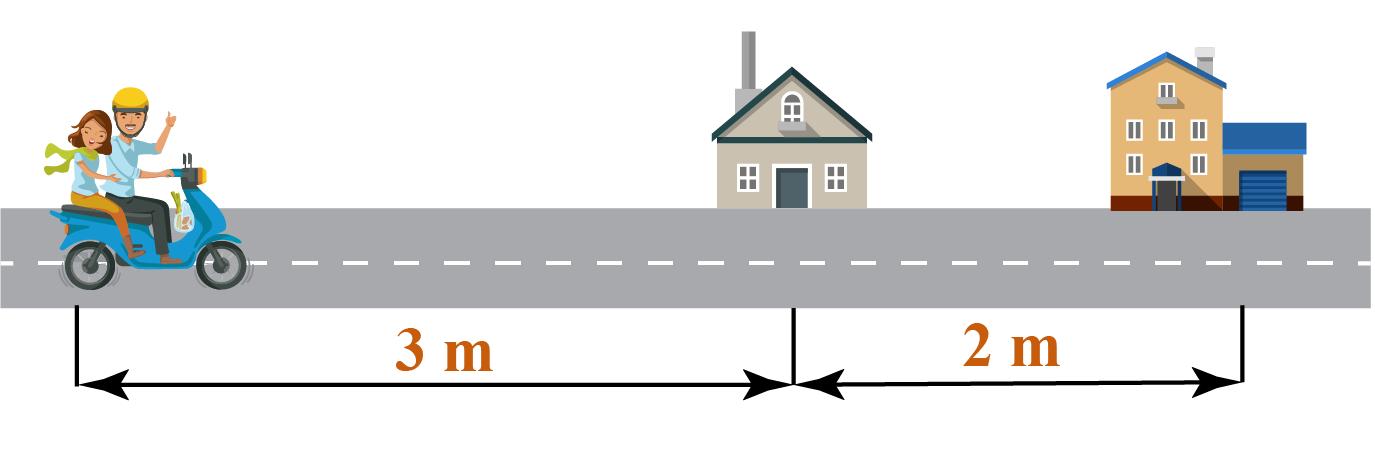We can do comparisons in terms of weights.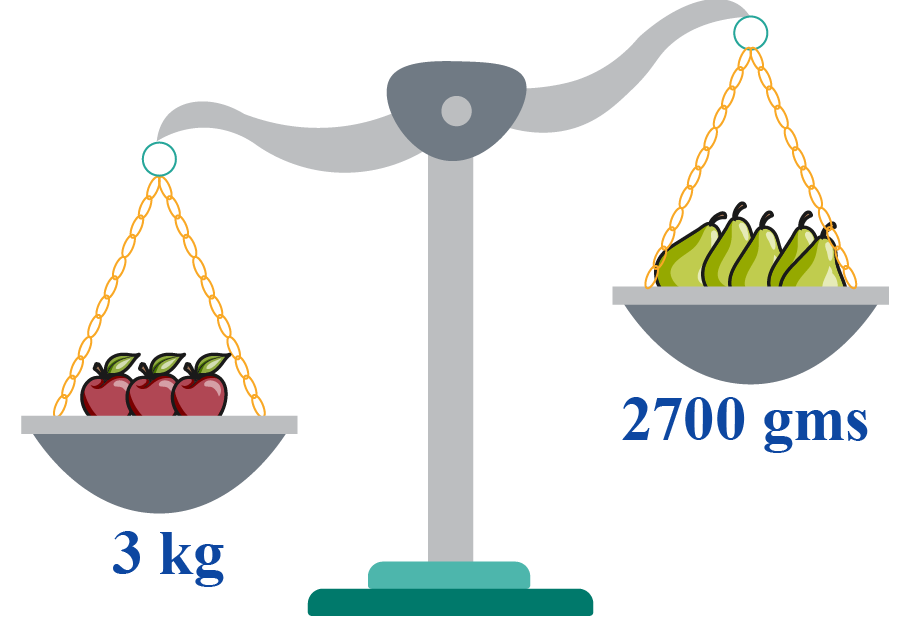We can do comparisons in terms of the volume of liquids.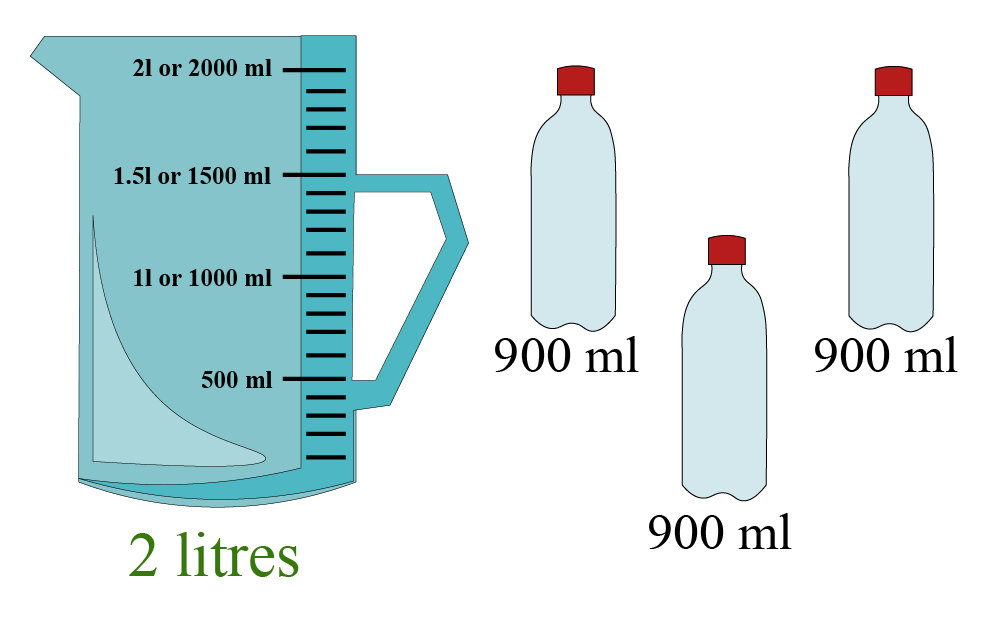We often make comparisons in our daily lives.

We view some things in relation to some other thing to make these comparisons.

In mathematics, we have important symbols or signs to understand bigger numbers, smaller numbers and equal numbers.

We use the following signs to compare numbers.

Signs   Example
> When one number is bigger than the other number, we put > sign between those numbers. $$9>5$$
= When two numbers are equal, we put = sign between those numbers. $$1+4=5$$
< When one number is smaller than the other number, we put < sign between those numbers. $$5<9$$

## What is Greater than Sign?

When we compare numbers, we are talking about their values.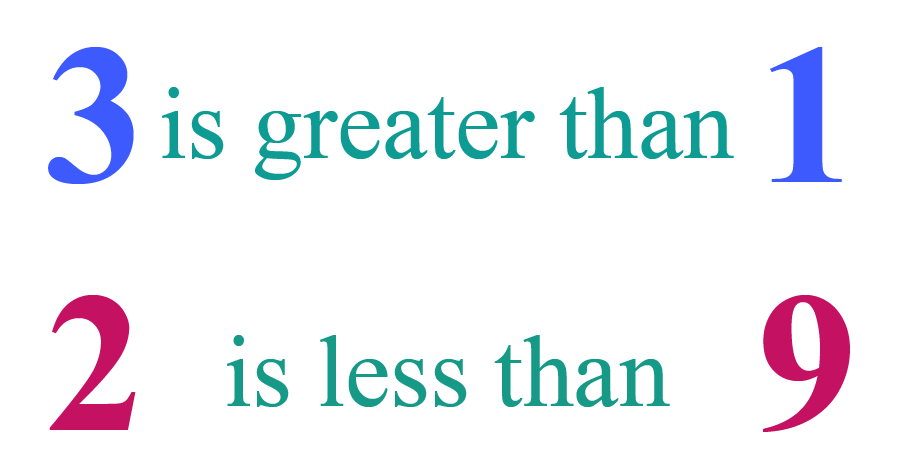Instead of using words, we use mathematical signs to understand their sizes.

Mathematical signs are recognised around the world because they make work quicker and simpler.

Have you seen this sign before?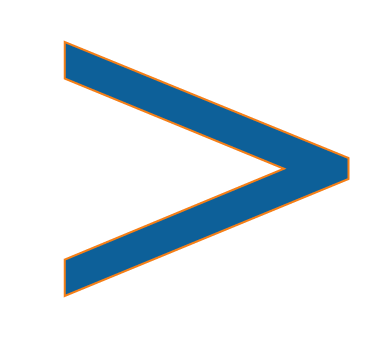This sign is called the greater than sign in math.

This is used to show that one value is greater than the other value.

We know that the statement "4 is greater than 2" is true.

Hence, we can write it as $$4>2$$

Now, let's understand the greater than sign from the left side to the right side.

On the left side of the sign, there are two points whereas on the right side, there is only one point.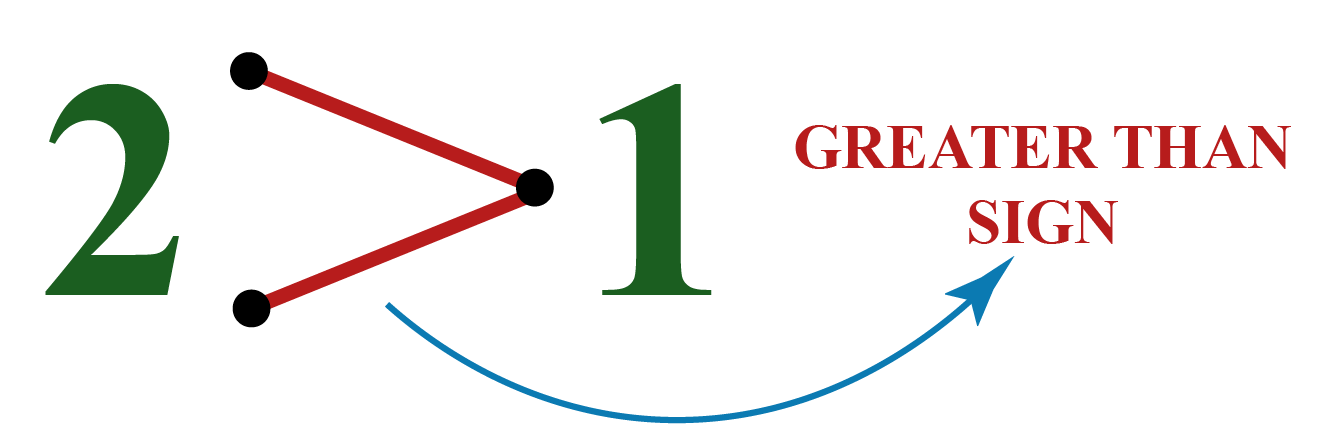Since two is greater than one, this is a greater than sign.

The wide open side of the sign having two points always faces the bigger number, whereas the smaller end point faces the smaller number.

Help your child score higher with Cuemath’s proprietary FREE Diagnostic Test. Get access to detailed reports, customised learning plans and a FREE counselling session. Attempt the test now.

## Greater than Sign: Examples

Let us learn a few comparison symbols: Greater than, Less than and Equal to.

We'll use the mouth of an alligator to represent these symbols.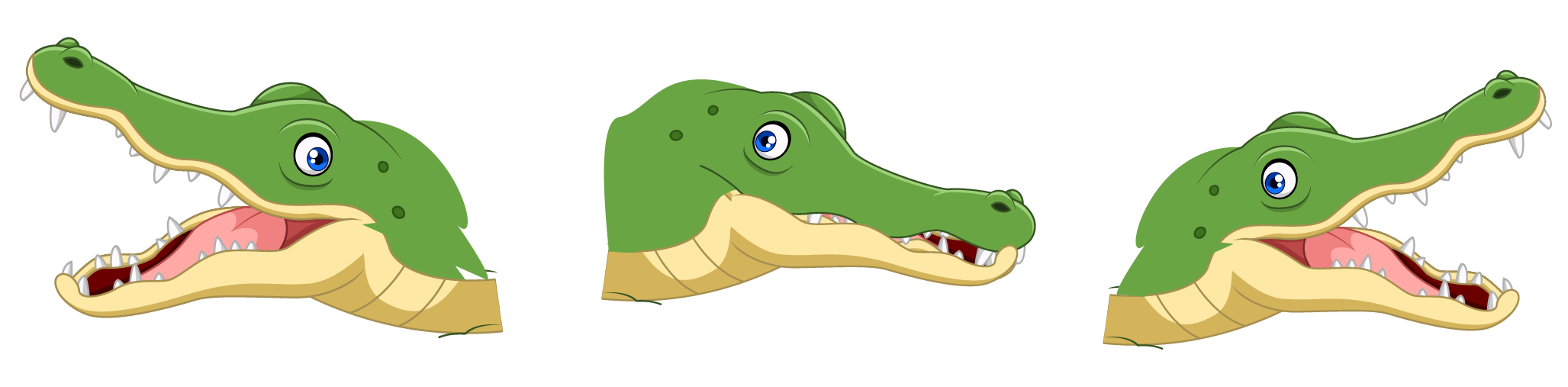You will have to remember that the mouth of this alligator is always open to the larger number.

 Example 1

On the left side, there are 6 stars and on the right side, there are 4 stars.

Insert the appropriate sign between the numbers.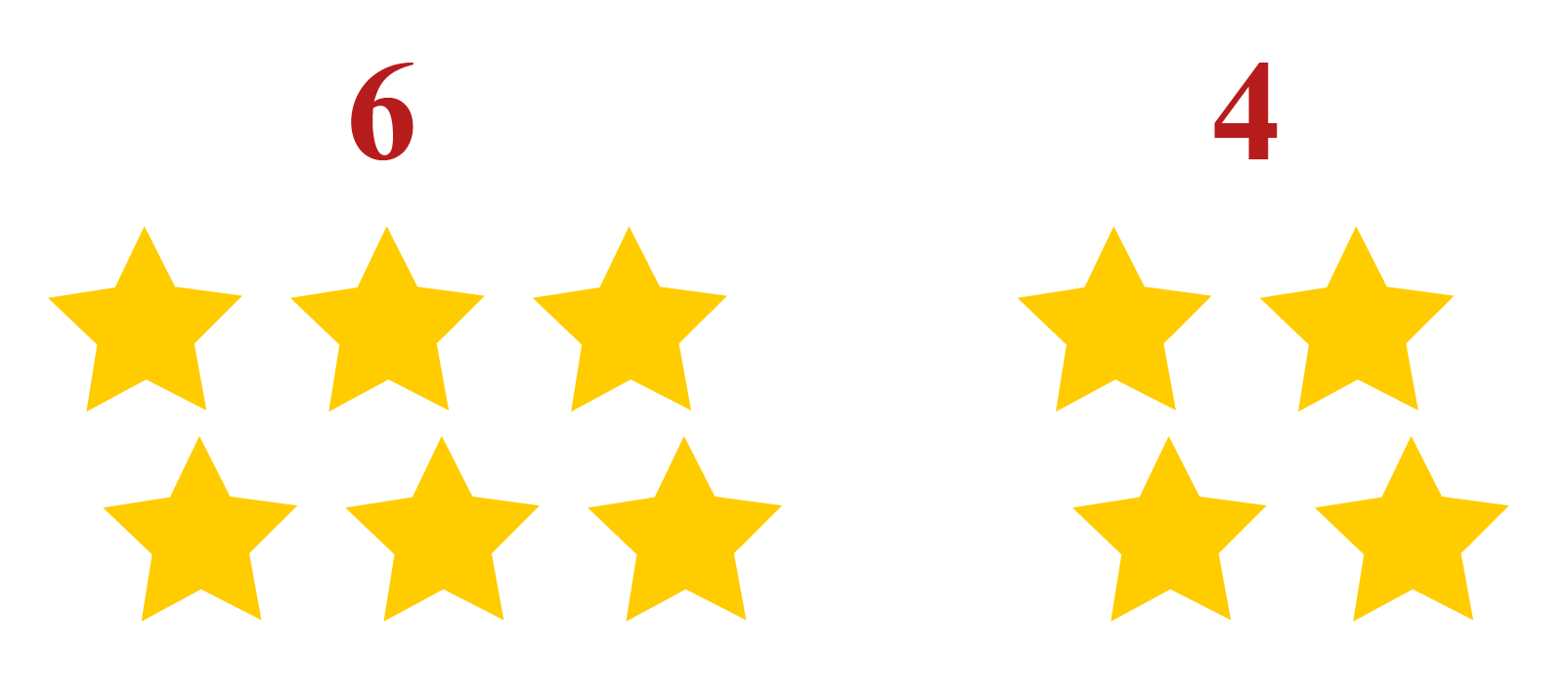Solution:

The number 6 is larger than the number 4.

Hence, the alligator's open mouth faces number 6.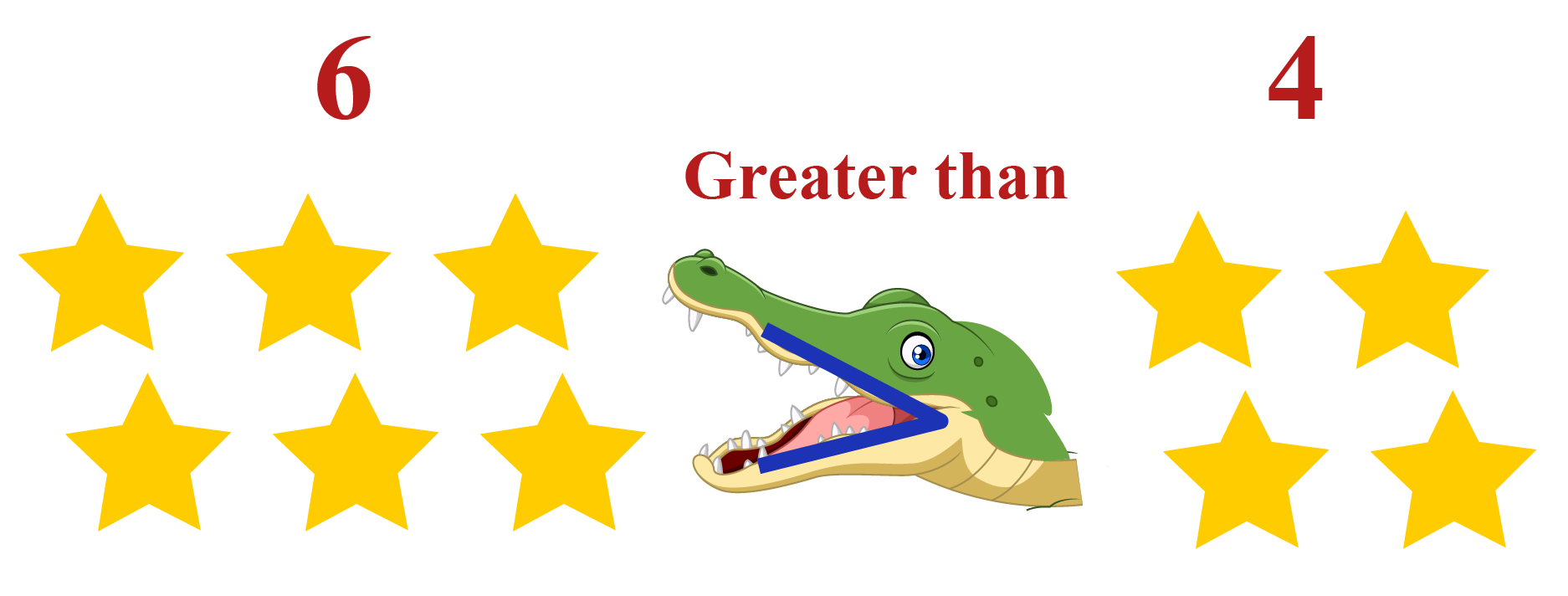Here, we can say that 6 is greater than 4.

 6 $$>$$ 4
 Example 2

Now, its time to take the symbols beyond the alligator's mouth.

Which sign should we use with these numbers?

8

Solution:

Let's put two points beside the larger number and one point beside the smaller number.

Join the points to form the correct sign.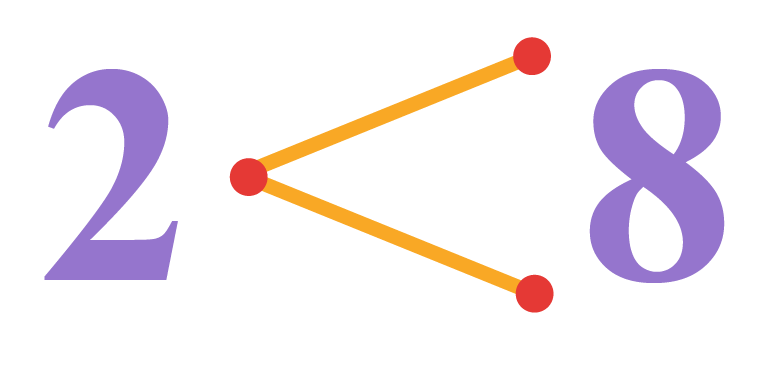This statement says that 2 is less than 8.

 2 $$<$$ 8

You can notice from these examples that writing the sign is easy.

But, reading it needs a bit more practice.

 Example 3

Harry was reading a newspaper.

Suddenly, a news article caught his attention.The news article was about the population of cities.

The population of Harry's city was 56,34,567 and the population of his neighbouring city was 7,34,193

Harry wonders which city is more populated.

Can you help him?

Solution:

Let's compare the population of both cities.

Count the number of digits in both the numbers and find out which number has more number of digits.

The number 56,34,567 has more digits than the number 7,34,193

We know that the whole number having more number of digits is greater than the whole number with less number of digits.

This means that Harry's city is more populated than his neighbouring city.

 56,34,567 $$>$$ 7,34,193Tips and Tricks

 1. One of the easiest ways to remember the "greater than" and "less than" signs is to imagine them as hungry alligators that always eat the larger number. Hence, the wide-open side of the sign faces the larger number.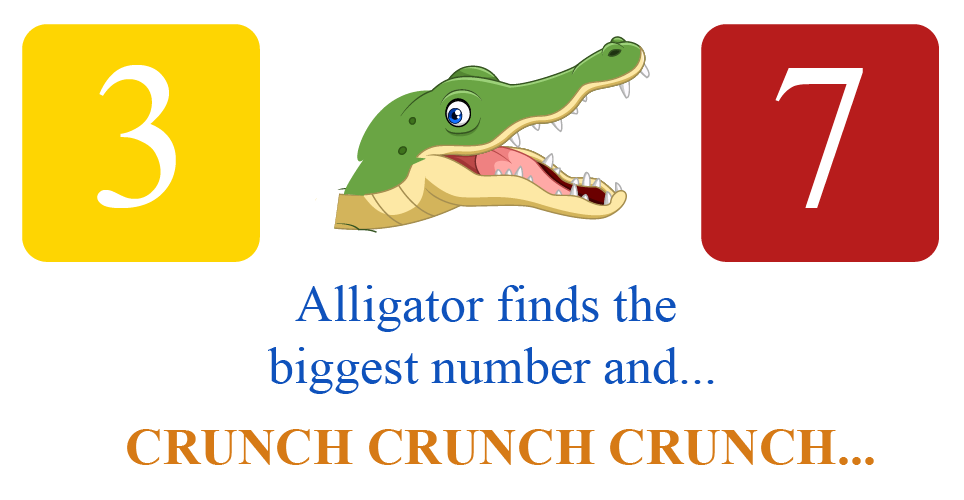2. Another way to memorise them is to observe that the sign "<" looks similar to the alphabet "L". In other words, "<" means "Less than".

## How to use the Greater than Sign in Math?

Let's compare two digit numbers, 32 and 14

While comparing the two-digit numbers, we first compare the tens digits.

If the tens digits are the same, we will compare the ones digits.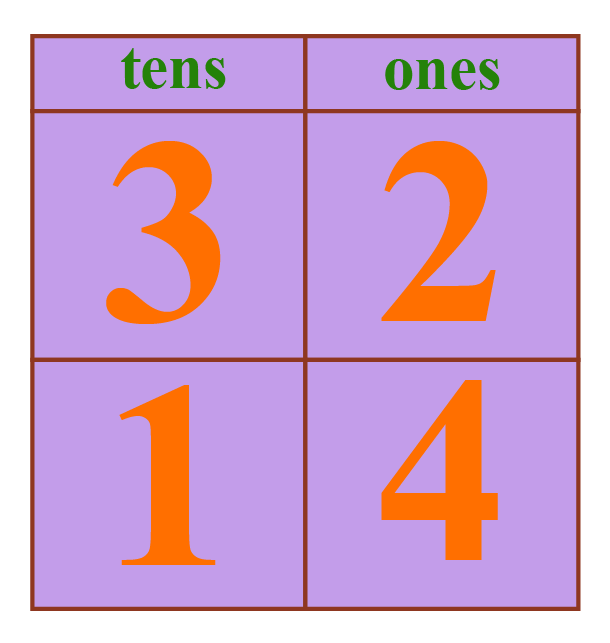Here, we can see that 3 is greater than 1

Thus, we can say that 32 is greater than 14

Mathematically, we can write it as $$32>14$$

 Example 1

Frank is working on an assignment for his class.

He has a list of countries which need to be arranged in an ascending order according to their annual wheat harvest.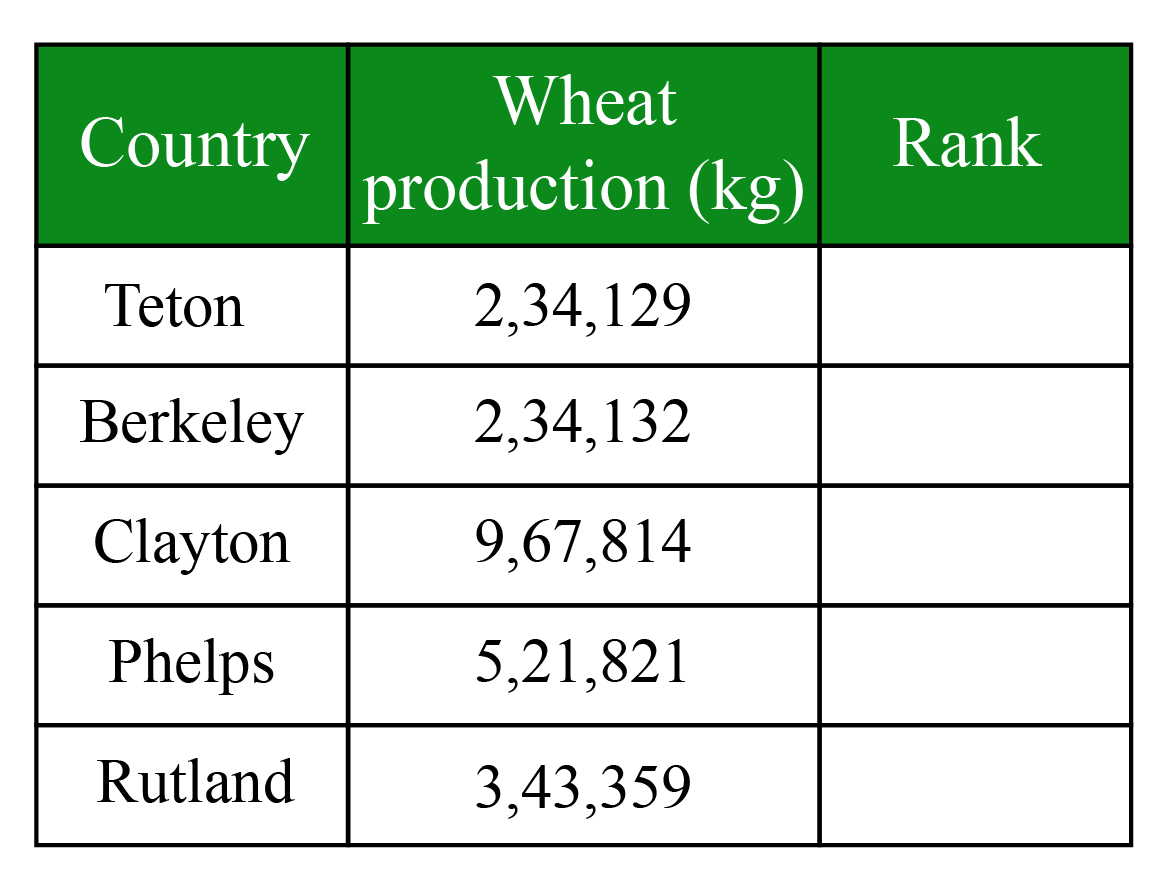Can you help him complete this task?

Solution:

Arranging the numbers in ascending order means to arrange them from the lowest to the highest.

You can notice that all the numbers are 6-digit numbers.

Hence, we will start comparing from the left-most digit in the number.

Starting from the first digit, we see that Clayton has the largest wheat production.

Thus, Clayton will be ranked 5th

Now, one of the countries among Teton and Berkeley has the lowest wheat production.

Since all the digits before the tens place are the same, we compare the tens digits.

Thus, Teton will be ranked 1st and Berkeley will be ranked 2nd

We are now left with two states: Phelps and Rutland.

Since the left-most digit of 3,43,359 is smaller than the left-most digit of 5,21,821, this means that Rutland will rank 3rd and Phelps will be ranked 4th

 2,34,129 $$<$$ 2,34,132 $$<$$ 3,43,359 $$<$$ 5,21,821 $$<$$ 9,67,814

### Greater than - Less than Calculator

One of the ways to compare the numbers is by using a number line.

Give two numbers and compare them using number line.

Hope you enjoyed this Greater than - Less than calculator!Think Tank
1. Write the following statement using mathematical symbols.

Five times a number $$x$$ is greater than the sum of 6 and a number $$y$$.
2. Make the greatest four digit number using the digits: 7, 0, 4 and 2 in such a way that no digit is repeated.

CLUEless in Math? Check out how CUEMATH Teachers will explain Greater than sign to your kid using interactive simulations & worksheets so they never have to memorise anything in Math again!

Explore Cuemath Live, Interactive & Personalised Online Classes to make your kid a Math Expert. Book a FREE trial class today!

## Practice Questions

Here are a few activities for you to practice.

Select/Type your answer and click the "Check Answer" button to see the result.

## Maths Olympiad Sample Papers

IMO (International Maths Olympiad) is a competitive exam in Mathematics conducted annually for school students. It encourages children to develop their math solving skills from a competition perspective.

You can download the FREE grade-wise sample papers from below:

To know more about the Maths Olympiad you can click here

## 1. What do you mean by the greater than symbol?

Greater than symbol is a mathematical symbol which is used when one value is greater than another value.

Greater than symbol looks like ">".

## 2. What is greater than or equal to symbol?

Greater than or equal to symbol is a mathematical symbol which is used when one value is either greater than or equal to some other value.

Greater than or equal to symbol looks like "$$\geq$$".

## 3. What are the examples of greater than symbols?

We know that the number 5 is greater than the number 2

Hence, we use the greater than symbol ">" to write it as "5 > 2"

More Important Topics
Numbers
Algebra
Geometry
Measurement
Money
Data
Trigonometry
Calculus